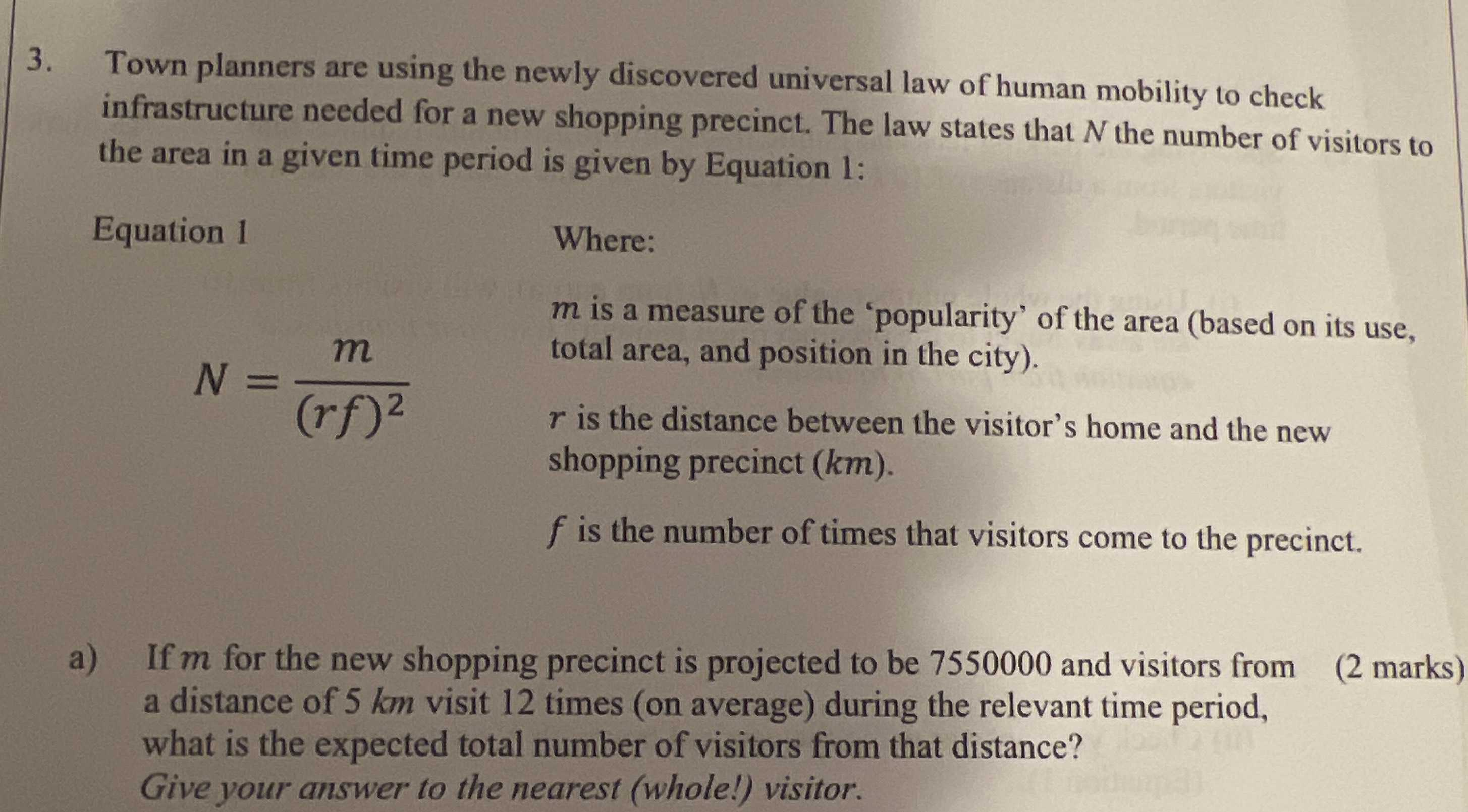### ¿Todavía tienes preguntas de matemáticas?

Pregunte a nuestros tutores expertos
Algebra
Pregunta3. Town planners are using the newly discovered universal law of human mobility to check infrastructure needed for a new shopping precinct. The law states that $$N$$ the number of visitors to the area in a given time period is given by Equation 1: Equation $$1$$:

Equation $$1$$ $$N = \frac { m } { ( r f ) ^ { 2 } }$$

$$m$$ is a measure of the 'popularity' of the area (based on its use, total area, and position in the city).

$$r$$ is the distance between the visitor's home and the new shopping precinct ( $$k m )$$

$$f$$ is the number of times that visitors come to the precinct.

a) If $$m$$ for the new shopping precinct is projected to be $$7550000$$ and visitors from a distance of $$5 km$$ visit $$12$$ times (on average) during the relevant time period, what is the expected total number of visitors from that distance? Give your answer to the nearest (whole!) visitor.